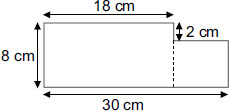# User ForumSubject :IMO    Class : Class 5

The given figure is made up of two rectangles.
Find its total area.A 144 cm2
B 216 cm2
C 240 cm2
D 300 cm2

There is a difference between square cm and cm squared (cm2). Most of your questions refer to square cm, but referred to as cm2. Please correct the same.

## Ans 1:

Class : Class 6
Bala Bala Shaitan Ka Saala

## Ans 2:

Class : Class 10
Area of rectangle ABCD = (18 × 8) cm2 = 144 cm2 total area = (144 72) cm2 = 216 cm2

## Ans 3:

Class : Class 6
Answer is b because the length of the bigger rectangle is 18 cm and breadth is 8 cm. The length of the smaller rectangle is 30 cm-18 cm=12 cm and breadth is 8 cm-2 cm=6 cm. The area of the bigger rectangle is 18 cm X 8 cm=144 sq. cm and the area area of the smaller rectangle is 12 cm X 6 cm=72 sq. cm. Area of the figure given is 144 sq. cm+72 sq. cm=216 sq. cm.